Publication date: 11/10/2021

## Power for One Sample Variance

Use the Power Explorer for One Sample Variance to determine a sample size for a hypothesis test about one variance. Select DOE > Sample Size Explorers > Power > Power for One Sample Variance. Explore the trade offs between sample size, power, significance, and the hypothesized difference to detect (defined as a ratio between the null and alternative hypothesis values). Sample size and power are associated with the following hypothesis test: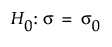versus the two-sided alternative: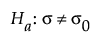or versus a one-sided alternative: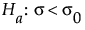or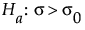where σ is the true variance and σ0 is the null variance or reference value. The difference to detect is an amount away from σ0 that one considers as important to detect based on a set of samples. This difference is expressed as the ratio of σ0/σa,or the ratio of your null variance and your assumed variance under the alternative hypothesis. For the same significance level and power, a larger sample size is needed to detect a small difference in variances than to detect a large difference. It is assumed that the population of interest is normally distributed with mean μ and standard deviation σ.

### Power Explorer for One Sample Variance Options

Set study assumptions and explore sample sizes using the radio buttons, text boxes, and sliders. The curve updates as you make changes to the settings. Alternatively, change settings by dragging the cross hairs on the curve or adjusting the values in the axis text boxes.

Test Type

Specifies a one or two-sided hypothesis test.

Fixed Parameters

Alpha

Specifies the probability of a type I error, which is the probability of rejecting the null hypothesis when it is true. It is commonly referred to as the significance level of the test. The default alpha level is 0.05.

Test Parameters

Parameters that are inter-related and update as you make changes.

Ratio of variances (Null/Alternative)

Specifies the ratio of the variance under the null hypothesis (reference variance) to the variance under the alternative hypothesis (expected variance).

Sample Size

Specifies the total number of observations (runs, experimental units, or samples) needed for your experiment.

Power

Specifies the probability of rejecting the null hypothesis when it is false. With all other parameters fixed, power increases as sample size increases.

Save Settings

Saves the current settings to the Saved Settings table. This enables you to save a set of alternative study plans. See Saved Settings in the Sample Size Explorers.

Make Data Collection Table

Creates a new data table that you can use for data collection.

### Statistical Details for the One Sample Variance Explorer

The power calculations for testing the variance of one sample group is based on the χ2 test. Calculations are based on the form of the alternative hypothesis.

For a one-sided, higher alternative: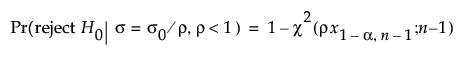for a one-sided, lower alternative: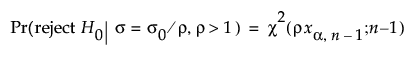for a two-sided alternative: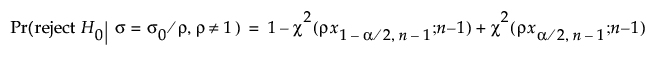where:

α is the significance level

n is the sample size

ρ=σ0a

x1-α,n is the (1 - α)th quantile of a central χ2 distribution with ν degrees of freedom

χ2(x, ν) is the cumulative distribution function of a central χ2 distribution with ν degrees of freedom.